# Overview of Trigonometry: Tutoring Solution Chapter Exam

Exam Instructions:

Choose your answers to the questions and click 'Next' to see the next set of questions. You can skip questions if you would like and come back to them later with the yellow "Go To First Skipped Question" button. When you have completed the practice exam, a green submit button will appear. Click it to see your results. Good luck!

### Page 1

#### Question 3 3. Solve the following trigonometric equation for θ.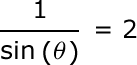#### Question 4 4. In the pictured triangle, side a is 9 and side b is 6. If ∠C is 26 degrees, then approximately how long is side c? Round the value of the cosine to the nearest whole number.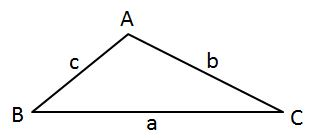#### Question 5 5. In the pictured triangle, side a is 23, side b is 18, and side c is 16. What is the approximate measure of ∠C? When calculating the inverse cosine, round the initial value to the nearest whole number.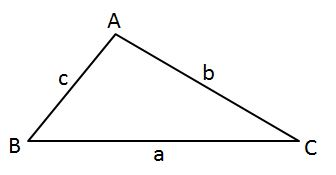### Page 2

#### Question 6 6. In the pictured triangle, side a is 32, side b is 24, and side c is 37. What is the approximate measure of ∠C? When calculating the inverse cosine, round the initial value to the nearest whole number.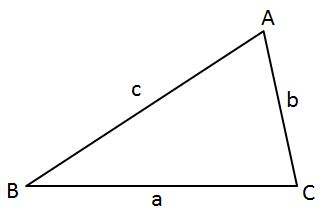#### Question 7 7. Solve for the value of x in the trigonometric equation below. (Use the domain -π/2 to π/2)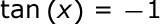#### Question 8 8. What is x?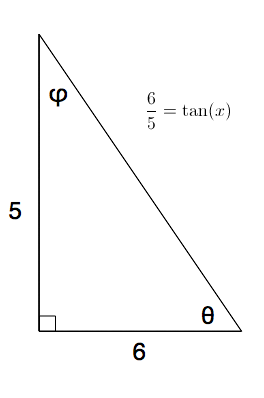### Page 3

#### Question 11 11. What's the value of sec2 (θ) given that the equation below is true?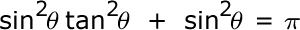#### Question 12 12. What is x?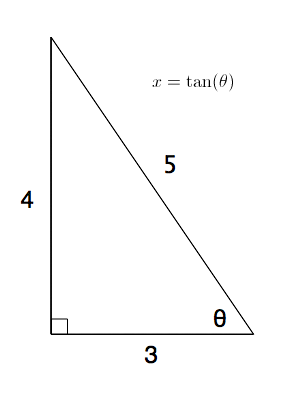#### Question 13 13. Simplify the following expression.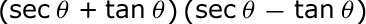#### Question 15 15. Convert the following measurement in radian to degrees.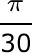### Page 4

#### Question 16 16. What is x?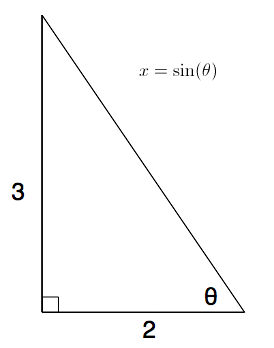#### Question 17 17. What is x?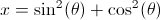#### Question 18 18. What is x?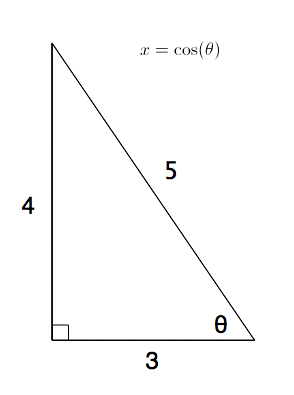#### Question 20 20. Perform the following calculation. Report the answer in radians.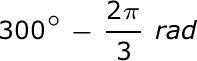### Page 5

#### Question 21 21. Simplify the following expression. Report the answer in degrees.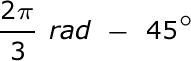#### Question 22 22. In the pictured triangle, side b is 12 and side c is 16. If ∠A is 84 degrees, then approximately how long is side a? Round the value of the cosine to the nearest whole number.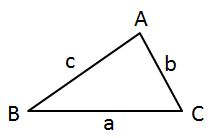#### Question 23 23. Solve the following equation for θ.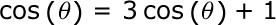#### Question 24 24. What is the following radian measure in degrees?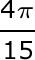#### Question 25 25. In the pictured triangle, ∠A is 137 degrees and ∠B is 28 degrees. If side b is 71 units long, approximately how long is side a?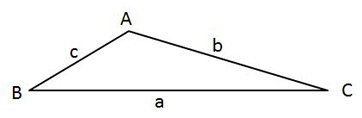### Page 6

#### Question 26 26. In the pictured triangle, ∠A is 58 degrees and ∠C is 63 degrees. If side a is 84 units long, approximately how long is side c?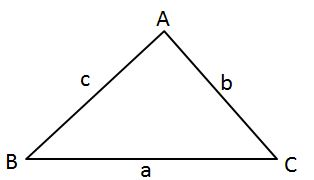#### Question 29 29. In the pictured triangle, ∠A is 62 degrees and ∠C is 86 degrees. If side a is 112 units long, approximately how long is side c?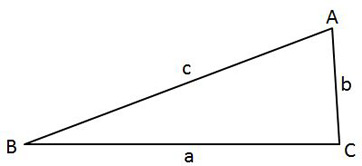#### Overview of Trigonometry: Tutoring Solution Chapter Exam Instructions

Choose your answers to the questions and click 'Next' to see the next set of questions. You can skip questions if you would like and come back to them later with the yellow "Go To First Skipped Question" button. When you have completed the practice exam, a green submit button will appear. Click it to see your results. Good luck!

Support